Home Math Computer Science Sport & Fun Photos

## Tim's Triangular Page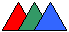This page may look rectangular but in fact it is a triangular page - just read the contents below and you will see.

A triangle is a closed plane figure bounded by three straight lines meeting at three different points. The three intersection points are triangle vertices. The line segments between the vertices are triangle sides. A triangle can also be defined as a three-sided polygon.

• The sum of the angles of a triangle is 180 degrees, and thus, every angle in a triangle is less than 180 degrees, and the biggest angle is at least 60 degrees.
• Every single side of a triangle is shorter than the sum of the lengths of the remaining two sides. The difference between the lengths of any two sides of a triangle is less than the length of the third side.
• An angle lying opposite to a bigger triangle side is also a bigger angle, and inversely.

#### Scalene Triangle

A triangle having three sides of different lengths is called a scalene triangle. All three angles of a scalene triangle are different. Inversely, if all three angles of a triangle are different, then this triangle is scalene.

#### Isosceles Triangle

A triangle having two sides of equal length is called an isosceles triangle. The two angles adjacent to the third side of an isoscales triangle are equal. If a triangle has two equal angles, it is an isoscales triangle, i.e. it has two equal sides too.

In an isosceles triangle, the median, bisectrix, and height of the vertext between the equal sides all coincide. This median/bisectrix/height divides the isoscales triangle into two congruent right triangles. If a median coincides with a height, or if a bisectrix coincides with a height, or if a median coincides with a bisectrix (see definitions below), then the triangle is isosceles - the adjacent sides are equal.

 If BD is a median and a height of triangle ABC, then triangles ABD and CBD are equal because they are right triangles whose both legs are equal, respectively. Therefore, AB = BC. If BD is a bisectrix and is also a height, then triangles ABD and CBD are equal because they are right triangles with one common leg and equal acute angles. If BD is a median and a bisectrix of triangle ABC, then triangles ABD and CBD are equal because all three angles of ABD and CBD are equal, respectively, and AD = CD.

The medians of the equal sides of an isosceles triangle are equal. The heights and bisectors crossing the equal sides are equal too (see definitions below).

 If AE and CD are medians of triangle ABC in which AB = BC, then triangles AEC and CDA are equal because they have a common side, AD = EC and angle BAC = BCA. Therefore, AE = CD. If AE and CD are heights of ABC, then right triangles AEC and CDA are equal because they have a common hypotenuse and angle BAC = BCA. If AE and CD are bisectors of triangle ABC, then triangles AEC and CDA are equal because angles BAC = BCA, ACD = CAE, and again they have a common side.#### Equilateral Triangle / Equiangular Triangle

A triangle having all three sides of equal length is called an equilateral triangle. A triangle having three equal angles is called an equiangular triangle.

The angles of an equilateral triangle are all the same, they all measure 60 degrees. Thus, every equilateral triangle is also an equiangular triangle. Invesely, every equiangular triangle is also an equilateral triangle.

The sum of the distances from any point in the interior of an equilateral triangle to all three sides is always equal to the height (see its definition below) of the equilateral triangle.

 Let us draw lines DE parallel to AC, FG parallel to AB, IJ parallel to BC. Triangles FPJ, DIP, and PGE are similar to ABC and therefore are equilateral. Denote the heights of the triangles FPJ, DIP, PGE, and ABC by e, f, g, h, respectively. e/h = FJ/AC, f/h = DI/AB, g/h = GE/BC. Let us add these thee equalities. Note that DP = AF and PE = JC because ADPF and JPEC are apallelograms. Using the fact that three sides of each of FPJ, DIP, PGE, and ABC are equal, we get the following equality: (e+f+g))/h = 1.#### Acute Triangle

A triangle having three acute angles, i.e. angles that measure less than 90 degrees, is called an acute triangle.#### Obtuse Triangle

A triangle having an obtuse angle, i.e. an angle that measures more than 90 degrees, is called an obtuse triangle. Note that the other two angles of an obtuse trianle are less than 90 degrees.#### Right Triangle

A triangle having a right angle, i.e. an angle that measures 90 degrees, is called a right triangle. Note that the other two angles of a right trianle are less than 90 degrees. The two acute angles of an isosceles right triangle measure 45 degrees.

The side opposite the right angle is called the hypotenuse. The two sides that form the right angle are called the legs. Every leg in every right triangle is shorter than the hypotenuse. The sum of the angles adjacent to the hypotenuse is 90 degrees. The distances between the midpoint of the hypotenuse and all three vertices are the same. In other words, the length of a hypotenuse median (see its definition below) is half of the hypotenuse length.

 Let us circumscribe a circle around right triangle ABC. If angle BAC is 90 degrees, then chord BC is a diameter and the midpoint M of BC is the center of the circumcircle. Thence, MA is also a radius of the circumcircle like MB and MC.#### Congruence

Two triangles are congruent (equal) if they have identical size and shape so that thet can be exactly superimposed.

Two congruent triangles ABC and CDA with a common side and equal angles located at two different ends of the common side always form a parallelogram. If AB=BC, then it is a rombuss. If angle ABC is 90 degrees, it is a rectangle. If AB=BC and angle ABC is 90 degrees, it is a square.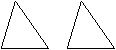#### Similar Triangles

Two triangles are called similar if all their angles are equal, respectively. Note that it is sufficient for two triangles to have two pairs of equal angles to be similar.

If triangles are congruent, then they are similar es well. In similar triangles, corresponding sides are proportional. Inversely, if all three sides of two triangles are proportional, then these triangles are similar. If two sides of two triangles are proportional and the angle between the sides are equal, then these triangles are similar.

If r is the ratio of sides of two similar triangles, then the ratio of their areas (see its definition below) is r2. In similar triangles, heights, bisectors, and medains (see definitions below), respectively, are proportional as well.

 This follows from the fact that each of the pairs of triangles formed by a height, bisector, or medain in one similar triangle is similar to its counterpart in the other. It is apparent that the angles of the triangles formed by heights and bisectors are equal, respectively. As far as the triangles formed by medians are concerned, two of their sides are apparently proportional, and the angles between the proportional sides are equal.

Consider triangle ABC. If D and E are the points of intersection of a line parallel to BC with sides AB and AC, respectively, then triangle ADE is similar to ABC. Inversely, if triangle ADE is similar to ABC, and D lies on AB, E lies on AC, then DE is parallel to BC.

If triangles ABC and ADE are such that AB and AE are segments of the same straight line, AC and AD are also segments of the same line, BC and DE are parallel, then triangles ABC and ADE are similar. In general, if two triangles have parallel (or coinciding) sides, respectively, then they are similar.

Consider right triangle ABC. If BD is a height going from the right-angle vertex, then triangles ABC, ABD, and BDC are all similar. In general, any line EF perpendicular to hypotenuse AC forms triangle AFE similar to ABC. The same would hold if E lied on BC.#### Outer (Exterior) angle

Angle BCD (and other angles like this) is called an outer (exterior) angle of triangle ABC. It is equal to the sum of both non-adjacent angles ABC and BAC. Therefore, the outer angle is bigger than each of the two non-adjacent angles of the triangle.#### Median, Centroid

The segment of the straight line joining a triangle vertex and the midpoint of the opposite site is called a median.

All three medians always intersect in one point called a centroid. This is a corollary of Ceva's theorem. Any centroid divides all medians into a 1:2 ratio, with the larger portion toward the vertex and the smaller portion toward the side: GD:AG=1:2, GE:BG=1:2, GF:CG=1:2.

 Let F be the median point of AB, FD be parallel to AC. Then, triangles ABC and FBD are similar, BD = BC/2, FD = AC/2. Triangles AGC and GDF are similar too. Therefore, GD = AG/2 and GF = CG/2. Same considerations about line FE drawn parallel to BC lead to the conclusion that EG = BG/2.In a isosceles triangle, equal sides have equal medians and inverseley. A median separates the triangle into two triangles of equal area. A longer side always has a shorter median.

 Let BC > AB. If CD and AE are medians, then DE is parallel to AC. Let us draw DF and EG perpendicular to AC. FDEG is a rectangular. ADF and GEC are right triangles whose legs DF and EG are equal. Since the hypotenuse of GEC is longer than the hypotenuse of ADF, leg GC is also longer than leg AF. (It follows from Pythagorean theorem.) In right triangles AEG and FDC, leg FC is longer than leg AG. The other legs are equal again. Therefore, hypotenuse CD is longer than hypotenuse AE.A triangle angle is acute, right, or obtuse if and only if half of the length of the opposite side is less, equal, or more than its median.

 If AC < 2*BM, then AM < BM and MC < BM. Therefore, angle ABM < BAM; MBC < BCM, ABC < BAC + BCA. Hence, angle ABC is less than 90 degrees. The cases AC = 2*BM and AC > 2*BM are similar.The following is called Midpoint Theorem. Consider a triangle whose vertices (D,E,F) are the three midpoints of a given triangle ABC. Triangle DEF is congruent to triangles AFE, BFD, and CED. The medians of DEF coincide with the medians of ABC. The following pairs of lines are parallel: AB and ED, AC and FD, BC and FE. Besides, triangle ABC is similar to DEF, AFE, BFD, and CED. The lengths of the sides of DEF, AFE, BFD, and CED are half of the lengths of the respective sides of ABC: FD=AC/2, FE=BC/2, ED=AB/2.

 If F is the midpoint of AB, then draw FD parallel to AC and draw FE parallel to BC. Triangles AFE and ABC are similar and so are triangles BFD and BAC. Thus AE is half of AC and BD is half of BC, i.e. D and E are the midpoints of their respective sides. Triangles BFD and FAE are equal since AF = FB and their both adjacent angles are equal too because they are formed by parallel lines. Similar considerations can be applied to triangles BFD and DEC. Triangle FDE is equal to EAF because they share one side and its adjacent angkles are formed by parallel lines too. Finally, P is the midpoint of FE because triangles FDP and EAP are equal.#### Bisectrix (Bisector)

The segment of the straight line bisecting a triangle angle and going from the angle vertex till the opposite side is called a bisectrix or bisector. The distance from any bisector point to one adjacent side is the same as the distance to the other.

All three bisectors always intersect in one point. It follows from Ceva's theorem. Any point of a bisectrix is equidistant from the sides of the angle. In a isosceles triangle, equal sides have equal bisectors and inverseley. A longer side always has a shorter bisector.

 Suppose CM and AN be bisectors, and BC > AB. Circumscribe a circle through points A, C, and N. This circle crosses MC at point K that lies inside the triangle because angle KAN = KCN. Angles KAN and KCN are inscribed angles with the same chord. Since angle BAC is bigger than ACB, angle KAN is smaller than BAN. Chord KC is bigger than chord AN because a bigger incribed angle has a bigger chord and KAC > ACN. Therefore, MC > AN.Any bisectrix divides the side opposite to the angel into segments proportional to the sides adjacent to the angle.

 If BD is a bisectrix, let us draw line AE parallel to the bisector BD. Since triangles AEC and DBC are similar, (AD+DC)/DC = (EB+BC)/BC. Due to the fact that AE and BD are parallel, the following equalities hold for angles: AEC = DBC, EAB = ABD. Therefore, AEB is an isosceles triangle: EB = AB. Substituting AB for EB in the first equality and opening brackets gives the following: AD/DC = AB/BC.In every triangle, a bisectrix alway lies between the height and median going from the same vertex. If BE is a bisectrix of a right angle, BD is its height, and BF is its median, than angles DBE and EBF are equal.

 Let BD, BE, and BF are the height, bisector, and median, respectively. The case of the height lying outside ABC is proved similarly to this one. Consider case BC < AB. The opposite case is similar to this one. If BC = AB, then BD, BE, and BF coincide. First, angle BCA > BAC. Angle ABD > CBD because they complement angles BCA and BAC to 90 degrees. Consequently, angle ABD > ABE, and E lies between D and A. Second, CE/EA = BC/AB < 1. Hence, CE < EA, and CE < CF, that is, F lies between E and A. If angle ABC is 90 degrees, then BF = FA, angle ABF = CAB. Both angle CBD and CAB complement angle BCA to 90 degrees, and hence, they are equal. So are angles DBE and EBF.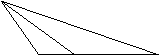#### Height (Altitude), Orthocenter

The segment of the straight line going from a triangle vertex till the opposite side (base) and crossing the opposite side or its continuation at the right angle is called a height or altitude.

In contrast to medians and bisectors which alway lie within their triangles, heights may lie outside of their triangles. It happens in obtuse triangles. Two their heights lie outsisde the triangle. In right triangle, two heights coincide with the legs. All three heights always intersect in one point called an orthocenter. Again, this is a corollary of Ceva's theorem. Orthocenters are located inside acute triangles and outside of obtuse triangles. In right triangles, orthocenters coincide with the vertices connecting two legs.

A longer side always has a shorter height. In a isosceles triangle, equal sides have equal heights and inverseley.

 If a, b, and c are triangle sides, and f, g, and h are their respective heights, then a*f = b*g = c*h because all three products amount to the doubled area of the triangle. Therefore, if a > b, then g > f, and so on.#### Incircle, Circumcircle, Euler Line

A circle that touches each of the triangle's three sides is called an inscribed circle or incircle. A circle that passes through each of the triangles three vertices is called a circumscribed circle or circumcircle. Both incircle and circumcircle are unique for every triangle.

The center of the incircle is located at the point of intersection of triangle bisectors. The center of the circumcircle is located at the point of intersection of three lines perpendicular to triangle sides and crossing them at the midpoints.

 The distances from the point of intersection of triangle bisectors to all three triangle sides are equal. Therefore, this point is the incircle center as well. Now, let F, G, and H are the midpoints of AB, BC, and AC, respectively. And let P be the point where perpendiculars going through F and G cross. Triangles AFP and FBP are equal (S-A-S), so are triangles BGP and GCP (Side-Angle-Side). Therefore, AP = BP and BP = CP. PH is perpendicular to AC because triangles APH and CPH are equal (Side-Side-Side) and angles AHP and CHP are bothe equal and complimentary.The center of the circumcircle of a right triangle is the midpoint of triangle's hypotenuse. The center of the circumcircle of acute triangles is located inside the triangles whereas it is located outside of obtuse triangles.

 The hypotenuse of a right triangle is a diameter of its circumcircle because its inscribed angle is 90 degrees. And the center of the circumcirle is the midpoint of the hypotenuse. Inversely, if the center of the circumcircle lies on a triangle side, then this is a right triangle. Let us prove the two following facts: - if the center of the circumcircle lies inside the triangle, then the triangle is acute - if the center of the circumcircle lies outside the triangle, then the triangle is obtuse These two facts along with the above fact about the circumcirles whose center is located on a triangle side imply that the center of the circumcircle of acute triangles is located inside the triangles and it is located outside of obtuse triangles. If D is the center of the circumcircle of triangle ABC, then angle EBC is 90 degrees. If D is inside triangle ABC, then angle ABC < EBC. If D is outside ABC, then angle ABC > EBC.The radius of the circumcircle of an equilateral triangle whose side is s equals s/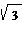whereas the radius of its incircle is s/(2*).

 If BE is a bisector and D is the point where medians, bisectors and heights of ABC intersect, then DE = BD/2. D is the center of the incircle. It is also the center of the circumcircle because the medians are perpendicular to the respective sides. By Pythagorean theorem BE =*s/2. The radius of the incircle is BE/3, the radius of the circumcircle is 2*BE/3.If an equilateral triangle is erected on each side of any triangle and these equilateral triangles are exterior to the original triangle, then the segments connecting the centers of the circumcircles of the three equilateral triangles form an equilateral triangle. This is called Napoleon's theorem. Click here to see a proof.

The orthocenter, centroid, and circumcenter of any triangle lie on the same line. It is called Euler line. Click here to see a proof. Another interesting circle related to triangles is the Nine-Point Circle. Click here to learn about the Nine-Point Circle.#### Perimeter, Area

The sum of the lengthes of all three triangle sides is called a perimeter. Half of a perimeter is called a semiperimeter. If point P is in the interior of triangle ABC, then AP + BP + CP is bigger than ABC semiperimeter (AB + BC + CA)/2.
 Since any triangle side is less than the sum of the other two, AB < AP + BP, AC < AP + CP, BC < BP + CP. Adding these three inequalities gives: AB + BC + AC < 2*(AP + BP +CP).

The area of a triangle is half of the product of its height and the base: (a.h)/2. Any of the three heights can be chosen for the calculation of the area. The result will be the same. The area of a right triangle can be calculated as half of the product of its legs. The area of a a equilateral triangle with side s equals*s2/4.

 If BE is a height, then it is also a median and EC = AC/2. By Pythagorean theorem BE =*s/2. The area of ABC is*s2/4.Heron's formula gives another expression for calculating the area. If p is the semiperimeter of a triangle, i.e. p = (a+b+c)/2, then the area is: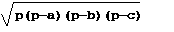#### Pythagorean Theorem

The sum of the areas of two squares whose sides equal to the two legs, respectively, of a right triangle is the same as the area of the square whose sides equal to the hypotenuse: a2+b2=c2. Click here to see a collection of various proofs of Pythagorean Theorem.

In every acute triangle, the area of the square whose sides are as the biggest triangle side is less than the sum of the areas of the two squares whose sides equal to two smaller sides, respectively. In every obtuse triangle, the area of the square whose sides are as the biggest triangle side is more than the sum of the areas of the two squares whose sides equal to two smaller sides, respectively.#### The Side-Side-Side Triangle Congruence Theorem

If two triangles have the same side lengths, then the triangles are congruent.

#### The Side-Angle-Side Triangle Congruence Theorem

If two triangles have two sides equal, respectively, and the angles between the two sides are equal too, then the triangles are congruent.

Corollary, if two right triangles have both legs equal, respectively, then they are congruent.

#### The Angle-Side-Angle Triangle Congruence Theorem

If two triangles have two angles and the sides between the two angles equal, respectively, then the triangles are congruent.

Corollary, if two right triangles have one leg and the adjacent acute angle equal, respectively, then they are congruent. If two right triangles have hypotenuse and one acute angle equal, respectively, then they are congruent.

#### No Angle-Side-Side Theorem!

Note that two triangles with two sides and a non-included angle equal are not necessarily congruent. In many instances, there could be two different triangles with given two sides and a non-included angle. See triangles ABD and ACD on the right.#### The Hypotenuse-Leg Triangle Congruence Theorem

If two right triangles have the hypotenuse and a leg equal, respectively, then the triangles are congruent.

#### Golden Triangle

An isosceles triangle such that the ratio of the length of the two equal sides to the length of the third side is the golden ratio is called a golden triangle. The angle between equal sides in a golden triangle is 36 degrees, which is 1/10 of the full angle or 1/5 of the straight angle. Note that the sides of the Great Pyramid of Giza are golden triangles.

When triangle study is over, it is time for a change. Why not to try puzzles now?

Copyright (c) 2004 Timothy Sakharov, Alexander Sakharov